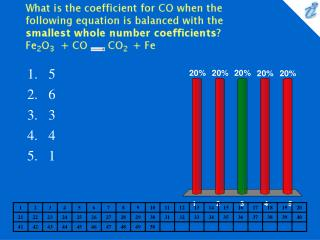DownloadDownload PresentationWhat is the coefficient for CO when the following equation is balanced with the smallest whole number coefficients? Fe2O

# What is the coefficient for CO when the following equation is balanced with the smallest whole number coefficients? Fe2O

Télécharger la présentation## What is the coefficient for CO when the following equation is balanced with the smallest whole number coefficients? Fe2O

- - - - - - - - - - - - - - - - - - - - - - - - - - - E N D - - - - - - - - - - - - - - - - - - - - - - - - - - -
##### Presentation Transcript

1. What is the coefficient for CO when the following equation is balanced with the smallest whole number coefficients? Fe2O3 + CO {image} CO2 + Fe • 5 • 6 • 3 • 4 • 1

2. Balance the following equation with the smallest whole number coefficients. Choose the answer that is the sum of the coefficients in the balanced equation. Do not forget coefficients of "one". C5H6NS + O2 {image} CO2 + H2O + NO2 + SO2 • 33 • 35 • 37 • 39 • 41

3. Balance the following equation with the smallest whole number coefficients. Choose the answer that is the sum of the coefficients in the balanced equation. Do not forget coefficients of "one". Cr2(SO4)3 + RbOH {image} Cr(OH)3 + Rb2SO4 • 10 • 12 • 13 • 14 • 15

4. How many moles of CO2would be produced from 56 moles of O2 according to the following balanced equation? 2C2H6 + 7O2 {image} 4CO2 + 6H2O • 16 • 32 • 224 • 48 • 8

5. What mass of SnCl4 must react with excess FeCl2 to produce 70.0 grams of SnCl2? SnCl4 + 2FeCl2 {image} SnCl2 + 2FeCl3 • 76.1 g • 96.2 g • 99.2 g • 106 g • 110 g

6. What is the maximum amount of Ca3(PO4)2 that can be prepared from 9.8 g of Ca(OH)2 and 9.8 g of H3PO4?3Ca(OH)2 + 2H3PO4 {image} Ca3(PO4)2+ 6H2O • 6.8 g • 8.6 g • 10.3 g • 13.7 g • 15.5 g

7. If a reaction of 27.5 g of Fe with 63.1 g Cl2produced 60.4 g of FeCl3, what was the limiting reactant and the percent yield? 3Cl2+ 2Fe {image} 2FeCl3 • Fe, 75.6% • Fe, 62.8% • Cl2, 41.8% • Cl2,63.0% • Fe, 37.8%

8. If 23.0 g of NaCl is dissolved in 300. g of H2O, what is the percent by mass of NaCl in the resulting solution? • 0.080% • 0.767% • 7.67% • 7.12% • 21.4%

9. The density of a 34.0% solution of NaBr is 1.33 g/mL. What volume of the solution contains 25.7 g of NaBr? • 101 mL • 6.57 mL • 32.8 mL • 105 mL • 56.8 mL

10. What mass of CaCl2 must be dissolved in enough water to produce 2000. mL of 1.25 M CaCl2? • 174 g • 277 g • 90.7 g • 81.1 g • 310 g

11. How many mL of 18.4 M H2SO4 are needed to prepare 600. mL of 0.10 M H2SO4? • 1.8 mL • 2.7 mL • 3.3 mL • 4.0 mL • 4.6 mL

12. What volume of 3.00 molar sulfuric acid, H2SO4, is required to react with 250. grams of calcium carbonate, CaCO3? CaCO3 + H2SO4 {image} CaSO4 + CO2+ H2O • 208 mL • 462 mL • 767 mL • 833 mL • 946 mL

13. What volume of 0.385 molar nitric acid, HNO3, is required to react with 48.0 mL of 0.0770 M calcium hydroxide, Ca(OH)2, according to the following equation? 2HNO3 + Ca(OH)2 {image} Ca(NO3)2 + 2H2O • 24.8 mL • 9.62 mL • 38.4 mL • 19.2 mL • 45.0 mL